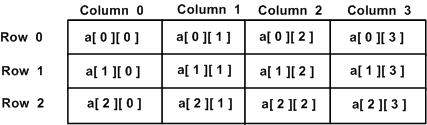# Go - Multidimensional Arrays in Go

Go programming language allows multidimensional arrays. Here is the general form of a multidimensional array declaration −

```var variable_name [SIZE1][SIZE2]...[SIZEN] variable_type
```

For example, the following declaration creates a three dimensional 5 . 10 . 4 integer array −

```var threedim int
```

## Two-Dimensional Arrays

A two-dimensional array is the simplest form of a multidimensional array. A two-dimensional array is, in essence, a list of one-dimensional arrays. To declare a two-dimensional integer array of size [x, y], you would write something as follows −

```var arrayName [ x ][ y ] variable_type
```

Where variable_type can be any valid Go data type and arrayName will be a valid Go identifier. A two-dimensional array can be think as a table which will have x number of rows and y number of columns. A 2-dimensional array a, which contains three rows and four columns can be shown as below −Thus, every element in the array a is identified by an element name of the form a[ i ][ j ], where a is the name of the array, and i and j are the subscripts that uniquely identify each element in a.

## Initializing Two-Dimensional Arrays

Multidimensional arrays may be initialized by specifying bracketed values for each row. Following is an array with 3 rows and each row has 4 columns.

```a = int{
{0, 1, 2, 3} ,   /*  initializers for row indexed by 0 */
{4, 5, 6, 7} ,   /*  initializers for row indexed by 1 */
{8, 9, 10, 11}   /*  initializers for row indexed by 2 */
}
```

## Accessing Two-Dimensional Array Elements

An element in two dimensional array is accessed by using the subscripts, i.e., row index and column index of the array. For example −

```int val = a
```

The above statement will take the 4th element from the 3rd row of the array. You can verify it in the above diagram. Let us check below program where we have used nested loop to handle a two dimensional array −

```package main

import "fmt"

func main() {
/* an array with 5 rows and 2 columns*/
var a = int{ {0,0}, {1,2}, {2,4}, {3,6},{4,8}}
var i, j int

/* output each array element's value */
for  i = 0; i < 5; i++ {
for j = 0; j < 2; j++ {
fmt.Printf("a[%d][%d] = %d\n", i,j, a[i][j] )
}
}
}
```

When the above code is compiled and executed, it produces the following result −

```a: 0
a: 0
a: 1
a: 2
a: 2
a: 4
a: 3
a: 6
a: 4
a: 8
```

As explained above, you can have arrays with any number of dimensions, although it is likely that most of the arrays you create will be of one or two dimensions.

go_arrays.htm